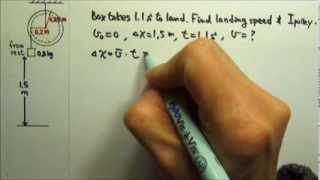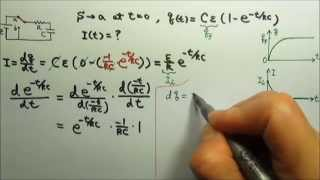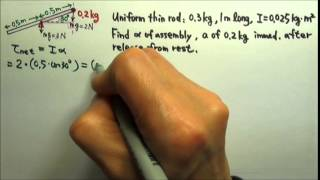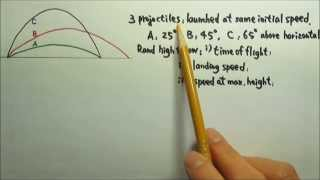## Yau Jong TwuYau Jong Twu Greenbelt, United States of America Yau-Jong Twu is a teacher at Eleanor Roosevelt High School in Greenbelt MD. She has been teaching the calculus-based AP Physics C since 1999 and the algebra-based AP Physics since 2003. Her AP Physics B / AP Physics 1 and 2 lesson videos are designed for students who have never had physics before. These videos are also used for "flipped classroom" teaching at Eleanor Roosevelt High School. Her AP Physics C lesson videos are designed for students who are at least taking pre-calculus concurrently. Please visit twuphysics.org for videos and supplemental material by topic.

## User videosRotation: Conservation of Energy Problem: pulley with mass
This lesson will look at a conservation of energy problem involving pulley with mass. This lesson will go through a step by step process to explain the concept. Using a diagram to give a look at the motion of the pulley to understand how to solve.DC Circuits: RC Circuits 4: Charging a Capacitor 3: Charging Current I
This lesson will look at an example question involving circuits. The given information will assist in solving for the components that need to be found. This lesson will draw diagrams to give a step by step explanation of how to derive the correct equation and better explain the concept.Rotation: Newton's Second Law for Rotation Problem 2
This lesson will look at an example question involving Newton's second law for rotation. The given information will assist in solving for the components that need to be found. This lesson will draw diagrams to give a step by step explanation of how to derive the correct equation and better explain the concept.Kinematics 29: Projectile Motion: Ranking Questions 2
This lesson will look at an example question involving kinematics. The given information will assist in solving projectile motion ranking questions. This lesson will draw diagrams to give a step by step explanation of how to derive the correct equation and better explain the concept.